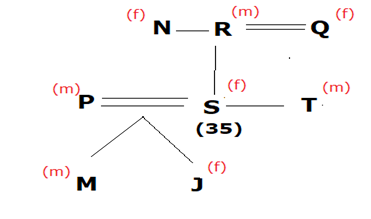# SBI Clerk Prelims Reasoning Ability Questions 2021 (Day-67)

Dear Aspirants, Our IBPS Guide team is providing new series of Reasoning Questions for SBI Clerk Prelims 2021 so the aspirants can practice it on a daily basis. These questions are framed by our skilled experts after understanding your needs thoroughly. Aspirants can practice these new series questions daily to familiarize with the exact exam pattern and make your preparation effective.

Start Quiz

Floor puzzle

Direction (1-5): Study the following information carefully and answer the questions given below:

There are eleven persons- A, B, C, D, E, F, G, H, I, J and K are living on eleven different floors but not necessarily in same order such that lowermost floor is numbered as one and just above it numbered as two and so on till the topmost floor is numbered as 11.

C lives on an even no. floor but above to fourth no. floor. Only three persons are living between D and C, who lives above to D. There are two floors between D and I, who is not adjacent to C’s floor. The number of persons living between D and E equal to persons living between D and B. E lives on an adjacent floor of I. K lives just above H and none of them lives on the tenth floor.A lives above C. Number of person between A and B are equal to the number of person between D and J. F lives above to G.

1) How many floors are there between F and K?

A.Three

B.Four

C.Five

D.Six

E.Two

2) As many persons live above B are equal to as many people’s lives below?

A.E

B.K

C.I

D.G

E.D

3) Which of the following is odd one?

A.GFD

B.EDG

C.HIK

D.JAC

E.FBJ

4) Who lives two floors above to G?

A.No one

B.The one who is just below C

C.The one who live two floors below J

D.The one live just below D

E.Both (c) and (d)

5) Which of the following is true?

A.J lives on an even no. floor

B.B lives just above D

C.E lives on third floor

D.C lives just above J

E.F lives two places below G

Order and ranking

Direction (6-8): Study the following information carefully and answer the questions given below:

There are eight trees- A, B, C, D, E, F, G and H of different heights. B is just shorter to F. Height of trees more than B is equal to no. of trees height less than E. There are three trees between E and C, which height is just shorter than B’s Height and Height of tree E is shorter than tree C. A is just taller to D. G’s is just taller to E. Tree which is of shortest height is 88cm and the third tallest oneis 166cm. Heights of all the trees are in natural number.

6) What can be the height of tree F?

A.167 cm

B.160 cm

C.169 cm

D.157 cm

E.140 cm

7) What can be the minimum possible sum of the height of tree B and E’s height?

A.254 cm

B.267 cm

C.256 cm

D.190 cm

E.280 cm

8) How many trees are shorter than H?

A.As many are taller than C

B.As many trees between A and C

C.two

D.Three

E.None of these

Blood relation

Direction (9-10): Study the following information carefully and answer the questions given below:

There are eight members i.e. J, M, N, P, Q, R, S and T in the family of three-generation. There are two married couples and three pairs of brother and Sister. Each couple has only two children.

P does not have any siblings. P is the son-in-law of R who is the brother of N. T is the uncle of M who is Son of S. Q is not married to P. P is the father of J and S age is 35.

9) If T’s and N’s age is more than S then how many persons are definitely older than S?

A.Four

B.Three

C.Two

D.Five

E.One

10) Who is the wife of P?

A.N

B.J

C.S

D.Cannot determine

E.Who is the sister of N

Directions (1-5) :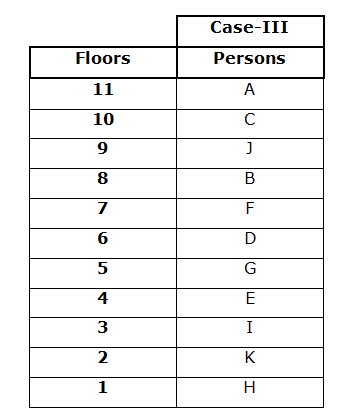We have,

• C lives on an even no. floor but above to fourth no. floor.
• Only three persons are living between D and C, who lives above to D.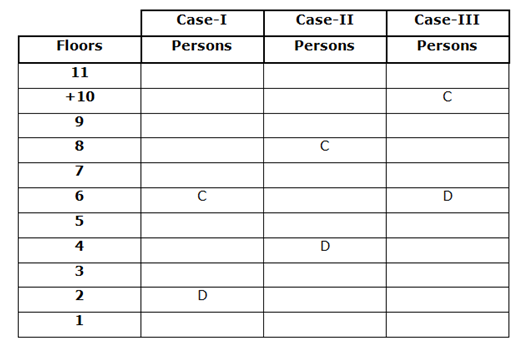• There are two floors between D and I, who is not adjacent to C’s floor.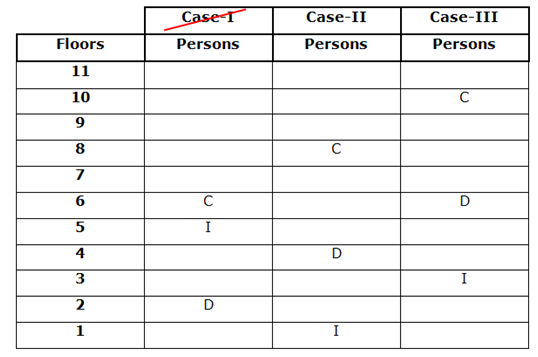Case-I Gets eliminated because I is not adjacent to C

• The number of persons living between D and E equal to the number of persons living between D and B.
• E lives on an adjacent floor of I.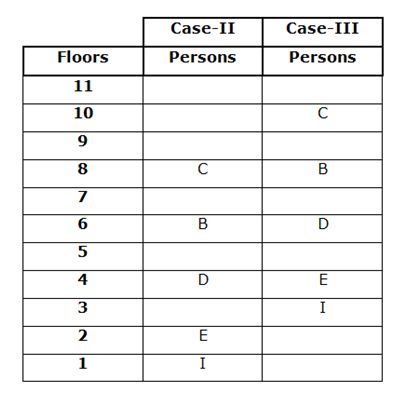• K lives just above H and none of them lives on the tenth floor.
• A lives above C. Number of persons between A and B are equal to the number of person between D and J.
• F lives above G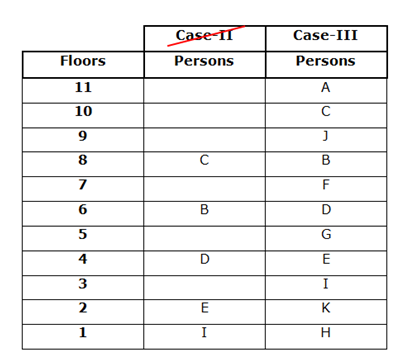Case-II gets eliminated because K and H cannot be on the 10th floor.

Directions (6-8) :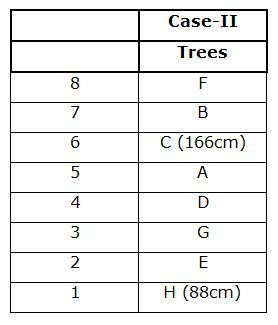We have,

• There are three trees between E and C, which is just shorter to B.
• Height of tree E is shorter than tree C.
• B is just shorter than F.
• Height of trees more than B is equal to no. of trees height less than E.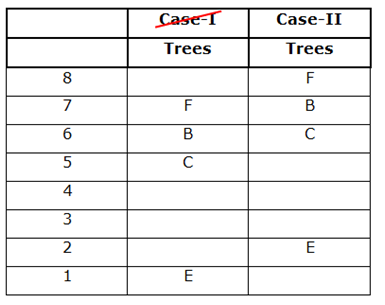• A is just taller to D. G is just taller to E.
• Tree which is shortest is 88cm and the one who is third tallest is 166cm.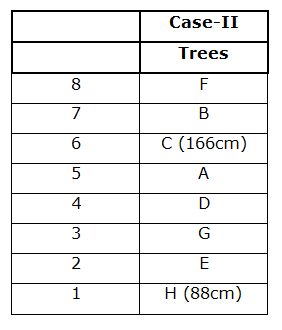Sum of Minimum possible heights of B and E= 167+89 = 256cm

Directions (9-10) :